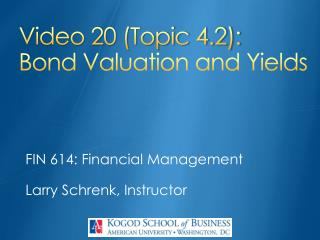DownloadDownload PresentationVideo 20 (Topic 4.2): Bond Valuation and Yields

# Video 20 (Topic 4.2): Bond Valuation and Yields

Download Presentation## Video 20 (Topic 4.2): Bond Valuation and Yields

- - - - - - - - - - - - - - - - - - - - - - - - - - - E N D - - - - - - - - - - - - - - - - - - - - - - - - - - -
##### Presentation Transcript

1. Video 20 (Topic 4.2):Bond Valuation and Yields FIN 614: Financial Management Larry Schrenk, Instructor

2. Topics • Discounted Cash Flow (DCF) Method • Bond Valuation • Bond Yields • Yield to Maturity (YTM) • Yield to Call (YTC) • Current Yield

3. Discounted Cash Flow (DCF) Method • Asset Value = PV(Cash Flows) • Examples: • Stock Price = PV(Dividends) • Project Value = PV(Net Annual Cash Flows)

4. Bond Valuation • Bond Value = PV(Cash Flows) • Two Cash Flows: • (Semi-Annual) Fixed Coupons • Par Value (at Maturity)

5. Bond Valuation • Bond Value = PV(Coupons) + PV(Par Value) • Coupons are an Annuity • Par Value is One Time Payment

6. Bond Valuation Formula for Bond Valuation PV(Coupons) PV(Par Value)

7. Bond Valuation: Example 1 What is the present value of a four year, semi-annual bond with a par value of \$1,000.00 and a coupon rate of 8% if the discount rate is 6%?

8. Bond Valuation: Example 2 What is the present value of a four year, semi-annual bond with a par value of \$1,000.00 and a coupon rate of 8% if the discount rate is 6%? N=8 (= 4 x 2) I%=6 PV=0 ◄ Select @, then [ALPHA] [ENTER] PMT=-40 (= (1000 x 0.08)/2) FV=-1000 P/Y=2 C/Y=2 PMT: ENDBEGIN PV = 1070.20 Note: Negatives

9. Yield to Maturity (YTM) • Discount rate such that Price = PV(cash flows) • Expectedreturn if the bond purchased at a fair value

10. Yield to Maturity: Example What is the YTM of a five year, semi-annual bond with a par value of \$1,000 and a coupon rate of 9% if the bond is selling for \$990? N=10 (= 5 x 2) I%=0◄ Select @, then [ALPHA] [ENTER] PV=-990 PMT=45 (= (1000 x 0.09)/2) FV=1000 P/Y=2 C/Y=2 PMT: END BEGIN PV (YTM) = 9.25% Note: Negatives

11. Yield to Maturity Notes • YTM = expected return only when just purchased. • YTM versus realized/actual yield

12. Yield to Call (YTC) • The yield of a bond if it is called, i.e., you were to buy and hold the security until the call date. • Calculation: Same as YTM except: • N = Periods to the Call Date (not Maturity) • FV = Call Price (not Par Value)

13. Yield to Call: Example What is the YTC of a five year, semi-annual bond with a par value of \$1,000 and a coupon rate of 9% if the bond is selling for \$990, the call price is \$1,100 and the call date is two years? N=4 (= 2 x 2; N is Periods to Call) I%=0 ◄ Select @, then [ALPHA] [ENTER] PV=-990 PMT=45 (= (1000 x 0.09)/2) FV=1100 (FV is Call Price) P/Y=2 C/Y=2 PMT: END BEGIN PV (YTM) = 14.09% Note: Negatives

14. Current Yield • Interest payment relative to price • Current Yield = Annual Interest Payment / Bond Price • It is not the bond’s expected return (that is YTM). • YTM = Current Yield + Capital Gains Yield

15. Current Yield: Example Find the current yield for a 9% annual coupon bond that sells for \$887 and has a par value of \$1,000. Current Yield = \$90 / \$887 = 0.1015 = 10.15%

16. Video 20 (Topic 4.2):Bond Valuation and Yields FIN 614: Financial Management Larry Schrenk, Instructor